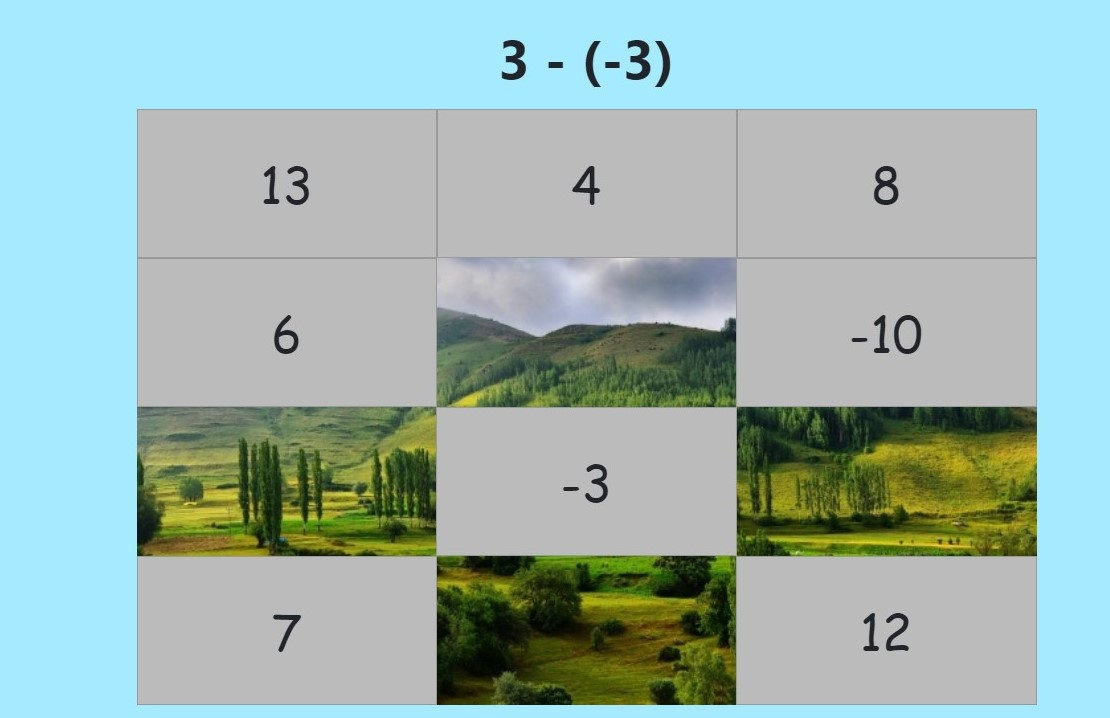MM Practice
×
Multiplication
Division
Place Value
Fractions & Decimals
Measurement
Statistics & Probability
Pre-Algebra
Money
Kindergarten
Geometry

# Integer addition & subtraction mystery picture gameOnline practice for 6th-9th grade

Solve integer addition and subtraction problems while uncovering a hidden picture in this fun game for middle school math!

You can choose the range of numbers to be used in the addition and subtraction problems, which means you can make the practice easy or difficult according to your need and grade level.

For example, choose a range from −10 to 10 for students that have just recently learned about integer addition and subtraction (6th and 7th grades). For a little more advanced practice, use −20 to 20, and for advanced level, −50 to 50.

Screenshot:"Correct" sound credit: http://www.orangefreesounds.com